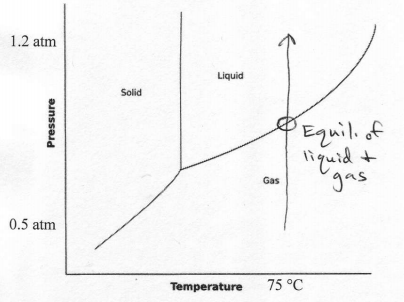# Problem: The phase diagram for a compound is shown below. Describe the phases and transitions as the pressure is increased from 0.5 atm to 1.2 atm at a constant temperature of 75 °C.

97% (85 ratings)
###### Problem Details

The phase diagram for a compound is shown below. Describe the phases and transitions as the pressure is increased from 0.5 atm to 1.2 atm at a constant temperature of 75 °C.What scientific concept do you need to know in order to solve this problem?

Our tutors have indicated that to solve this problem you will need to apply the Phase Diagram concept. You can view video lessons to learn Phase Diagram. Or if you need more Phase Diagram practice, you can also practice Phase Diagram practice problems.

What is the difficulty of this problem?

Our tutors rated the difficulty ofThe phase diagram for a compound is shown below. Describe th...as low difficulty.

How long does this problem take to solve?

Our expert Chemistry tutor, Sabrina took 2 minutes and 24 seconds to solve this problem. You can follow their steps in the video explanation above.

What professor is this problem relevant for?

Based on our data, we think this problem is relevant for Professor Gary's class at HAWAII.